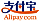Bookcover of A Study on Some Nonlinear Evolution Equations
Booktitle:

# A Study on Some Nonlinear Evolution Equations

LAP LAMBERT Academic Publishing (2016-09-20 )eligible for voucher
ISBN-13:

### 978-3-659-95623-2

ISBN-10:
3659956236
EAN:
9783659956232
Book language:
English
Blurb/Shorttext:
The book deals with nonlinear evolution equations (NLEEs) in in a unified direction. In generating new nonlinear equations, the connection between quantum mechanics and NLEEs is highlighted. The conventional Korteweg-de Vries (KdV) equation is connected with a time independent constant mass (CM) Schr¨odinger equation which describes the motion of a quantum mechanical particle of constant mass m in the presence of the potential V . We use the concept of generalized Schr¨odinger equation from quantum mechanics such as position-dependent mass Schr¨odinger equation or time-dependent mass Schr¨odinger equation and using Lax pair formulation, generate new generalized KdV equation. Such generalized KdV equation possesses variable coefficient term and called as inhomogeneous KdV equation. We apply inverse scattering transform (IST) method and show that such a generalized KdV equation still possesses soliton solution. For a particular choice of mass function, we explicitly obtain mass-deformed soliton solutions. The distinct difference between conventional KdV solitons and those for generalized KdV equation is noted and illustrated by interesting figures.
Publishing house:
LAP LAMBERT Academic Publishing
Website:
https://www.lap-publishing.com/
By (author) :
Amiya Das
Number of pages:
212
Published on:
2016-09-20
Stock:
Available
Category:
Mathematics
Price:
69.90 €
Keywords:
dynamical system, inverse scattering transform, Nonlinear evolution equations, soliton theory### LinksLOGIN
0 products in the shopping cart
Edit cartLOADING